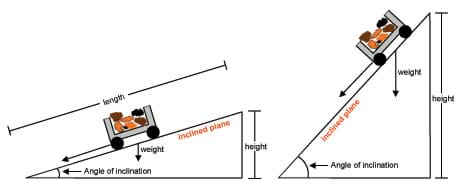Circuits for Four by Four

Automatic dark detector senses darkness.

modified from http://www.buildcircuit.com/darklight-sensor-using-transistor/

and

http://www.instructables.com/id/LED-nightlight-that-turns-on-in-darkness/

(1 ) As the light level decreases and LDR meets the maximum threshold resistance, the circuit automatically switches on the LED D1.  For more information download the file attached:

Automatic dark detector senses darkness

Videos – Step by step how to set up the circuit:

Lights up when there is light.

Inclined Plane 17/10

Forces acting on an object on an inclined planeThe mechanical advantage of a machine is the ratio of the load to the applied force. In other words, mechanical advantage determines how much force we need to perform a task. For example, the greater the mechanical advantage of a machine, the less force we need to have to perform a task such as moving an object. The opposite is true as well. Mathematically, mechanical advantage (MA) = load ÷ applied force. A good mechanical advantage is one that is greater than 1.

Inclined Plane

The purpose of an inclined plane as a simple machine is to move something from a lower height to a higher height with less effort. An object simply placed on a tilted surface often slides down the surface (see Figure 1) because of the force in the downhill direction. In other words, the forces in this scenario are unbalanced (i.e. there is no upward force to counteract the downward force and therefore, the object would slide down).The rate at which the object slides down is dependent upon how tilted the surface is; the greater the tilt of the surface, the faster the rate at which the object will slide down it. This is measured by the angle of inclination. Students can find this using a protractor. Friction also affects the movement of an object on a slope. Friction is a force that offers resistance to movement when one object is in contact with another. Imagine now that you were on the downside of the object and applying force to keep the object in the same place (not moving). To keep the object stationary, the force you would have to apply would need to equal the downward force due to gravity. That would be an example of balanced forces. If you wanted to push the force upwards, you would need to exceed the force of gravity.Figure 1: This diagram shows how ancient cultures used inclined planes to move heavy stones to the top of their pyramids. The force of gravity, friction and the pull force all affect how easy (or hard) it is to pull the cart up the inclined plane. copyright

To understand an object’s motion on an inclined plane, it is important to analyze the forces acting upon it. The force of gravity (also known as weight) acts in a downward direction. When the angle of inclination is greater, and the slope is steeper there is more weight component to overcome. With a shallower slope the weight component is easier to overcome and requires less effort.

The mechanical advantage of an inclined plane depends upon its slope and height. To find the mechanical advantage of an inclined plane, divide the length of the slope by its height.

Mechanical advantage of an inclined plane = length of slope ÷ height of plane

An inclined plane produces a mechanical advantage to decrease the amount of force needed to move an object to a certain height; it also increases the distance the object must move. The object moving up an inclined plane needs to move the entire length of the slope of the plane to move the distance of the height. For example, if you have a ramp with a slope length 20 meters that rises 5 meters high, then your trade-off is moving the 20 meters distance versus lifting straight up 5 meters, and your mechanical advantage is 4.

Screw